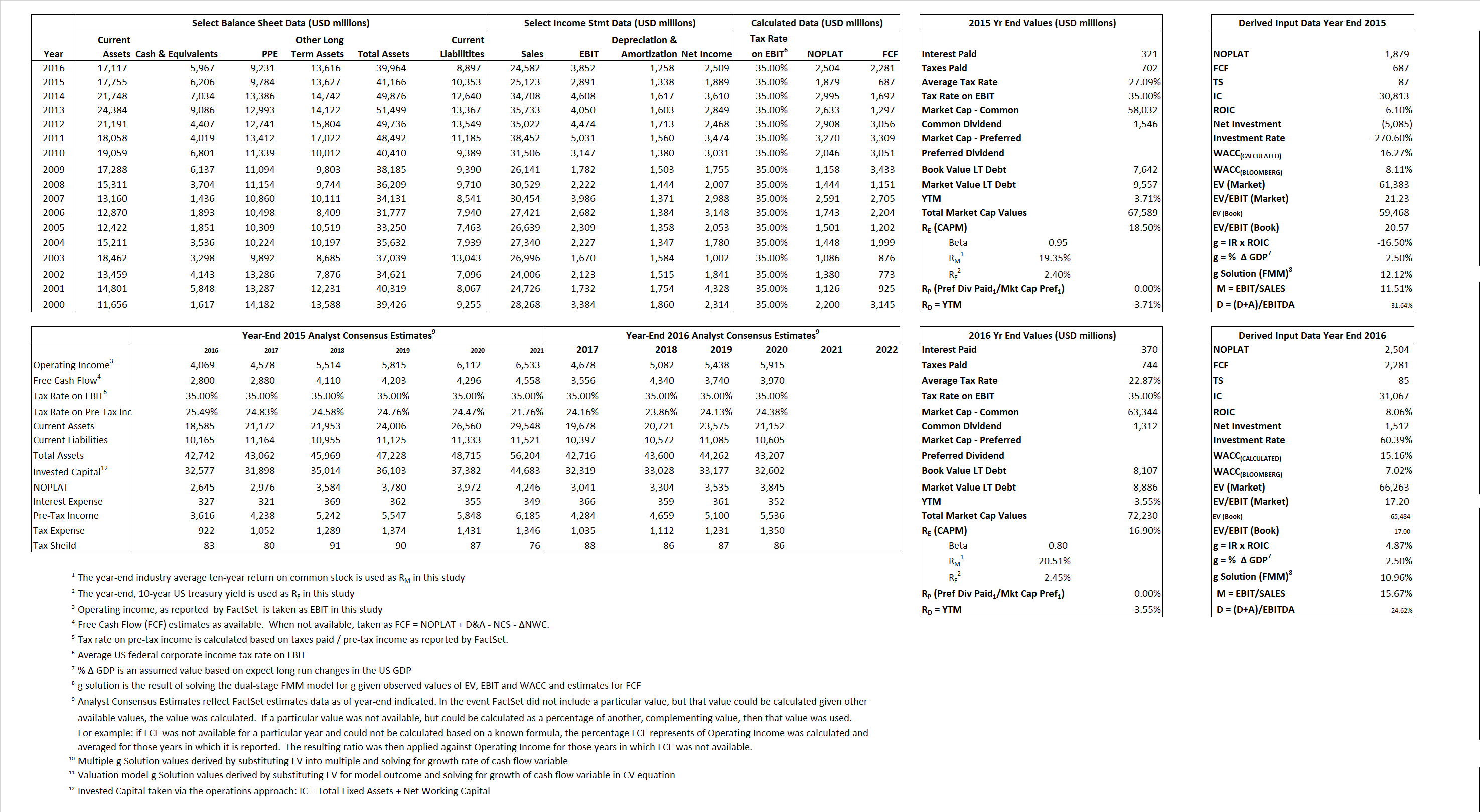# Du Pont

## Analyst Listing

The following analysts provide coverage for the subject firm as of May 2016:

 Broker Analyst Analyst Email Cowen & Company Colby Synesael colby.synesael@cowen.com Burke and Quick Partners, LLC Frederick W. Moran fmoran@bqpartners.com RBC Capital Markets Jonathan Atkin jonathan.atkin@rbccm.com Jefferies Jonathan Petersen jpetersen@jefferies.com Evercore ISI Jonathan Schildkraut schildkraut@evercoreisi.com Stifel Nicolaus Matthew Heinz heinzm@stifel.com

## Primary Input Data## Derived Input Data

### Equational Form

Net Operating Profit Less Adjusted Taxes NOPLAT 1,879  2,504$NOPLAT\, =\, EBIT\, x\, (1 \,-\, Avg \,\,Tax\,\, Rate\,\, on\,\, EBIT)$
Free Cash Flow FCF 687 2,281$FCF\,=NOPLAT\,+\,Non-Cash\,Expenses-\Delta NWC\,-\,NCS$
Tax Shield TS 87 85$TS\,=\,Interest\,\,Paid\,\,x\,\, Avg \,\,Tax\,\,Rate\,\, on\,\, Pre-Tax\,\, Income$
Invested Capital IC 30,813  31,067$IC\,=\,Fixed\,\,Operating\,\,Assets\,\,+\,\,Net\,\, Working\,\, Capital$
Return on Invested Capital ROIC 6.10% 8.06%$ROIC\,=\,\frac { NOPLAT }{ IC }$
Net Investment NetInv (5,085) 1,512$NetInv\,=\,{ {IC}_{1}}-{{IC}_{0}}+Depreciation$
Investment Rate IR  -270.60%  60.39%$IR\,=\,\frac {NetInv}{NOPLAT}$
Weighted Average Cost of Capital
WACCMarket 16.27%  15.16%$WACC\,=\,\frac { E }{ V } { R }_{ E }\,+\,\frac { P }{ V } { R }_{ P }\,+\,\frac { D }{ V } { R }_{ D }\left( 1- Avg\,\, Tax\,\,Rate\,\,on\,\,Pre-Tax\,\,Income \right)$
WACCBook     8.11%    7.02%
Enterprise value
EVMarket 61,383  66,263$EV\,=\,Market\,\,Cap\,\,Equity\,+\,\,Long\,\,Term\,\,Debt\,-\,Cash$
EVBook    59,468   65,484
Long-Run Growth
g = IR x ROIC
-16.50%    4.87% Long-run growth rates of the income variable are used in the Continuing Value portion of the valuation models.
g = %$\Delta$ GDP     2.50%    2.50%
Margin from Operations M    11.51%  15.67%$M\,\,=\,\,\frac{EBIT}{SALES}$
Depreciation/Amortization Rate D   31.64%  24.62%$D\,\,=\,\,\frac{D+A}{EBITDA}$

## Valuation Multiple Outcomes

The outcomes presented in this study are the result of original input data, derived data, and synthesized inputs.

### model g solution

12/31/2015 12/31/2016 12/31/2015 12/31/2016 12/31/2015 12/31/2016

EV/SALES$\frac {EV}{Sales} \,= \,\frac{ROIC\, -\, g}{ROIC\,(WACC\,-\,g)}\,(1\,-\,T)\,(M)$

2.44  2.70  26.52%  21.41%  20.43%  17.74%

EV/EBITDA$\frac {EV}{EBITDA} \,= \,\frac{ROIC\, -\, g}{ROIC\,(WACC\,-\,g)}\,(1\,-\,T)\,(1\,-\,D)$

14.51 12.97 26.52% 21.41% 20.43% 17.74%

EV/NOPLAT$\frac {EV}{NOPLAT} \,= \,\frac{ROIC\, -\, g}{ROIC\,(WACC\,-\,g)}$

32.67 26.47 26.52% 21.41% 20.43% 17.74%

EV/FCFOPS$\frac {EV}{FCF_{OPS}} \,= \,\frac{ROIC\, -\, g}{ROIC\,(WACC\,-\,g)}\,(1\,-\,T)$

89.35 29.05 26.52% 21.41% 20.43% 17.74%

EV/EBIT$\frac {EV}{EBIT} \,= \,\frac{ROIC\, -\, g}{ROIC\,(WACC\,-\,g)}\,(1\,-\,T)$

21.23 17.20 26.52% 21.41% 20.43% 17.74%

EV/IC$\frac {EV}{IC} \,= \,\frac{ROIC\, -\, g}{WACC\,-\,g}$

1.99 2.13 26.52% 21.41% 20.43% 17.74%